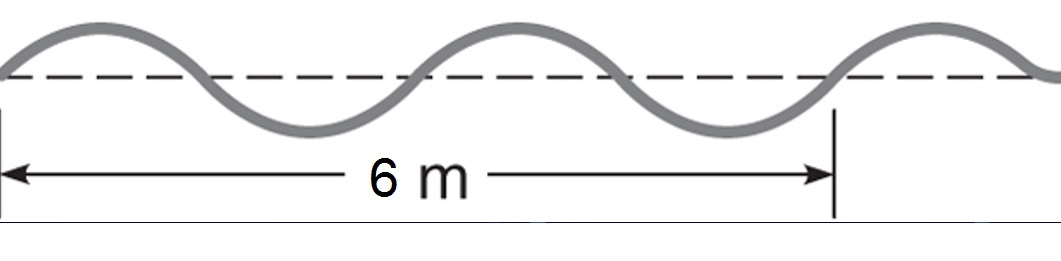# Quiz on Waves

Question 1: Which one of the following waves is a longitudinal wave?
• Water waves
• Sound waves
• Electromagnetic waves
• None of the above.
Question 2: What is the period of an ultrasound in a tissue in which the speed of sound is 1600 m/s
and the wavelength of the scanner is 4× 10− 4 m.
• 2.5 10− 7 s
• 2.5 10− 6 s
• 3.5 10− 7 s
• 2.5 10− 8 s
Question 3: If the speed of a wave remains constant, what happens to the frequency when the wave length is halved?

• Doubled
• Halved
• Same
• Zero
Question 4: Based on the wave below having a frequency of 50 Hz, find its speed.• 200 m/s
• 300 m/s
• 350 m/s
• 400 m/s
• Question 5: The vibrations in a transvers wave move in a direction ________________ to the wave motion.
• parallel
• perpendicular
• opposite
• upward
• downward